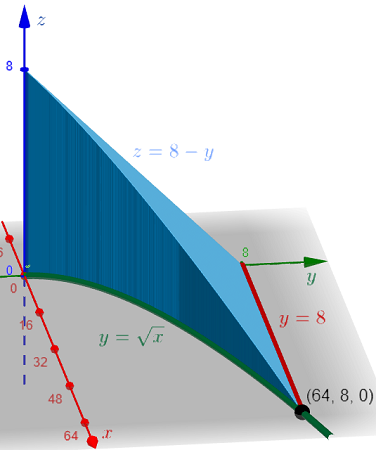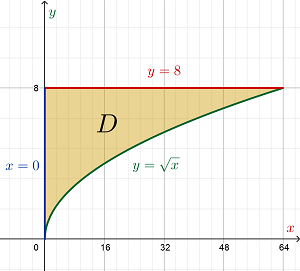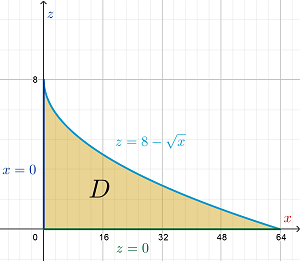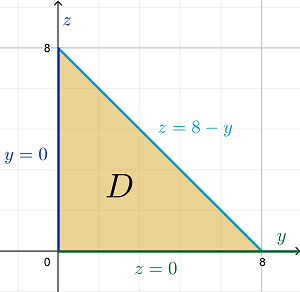# Rewrite the integral \int_0^{64} \int_{\sqrt x}^8 \int_0^{8-y} f(x,y,z) \,dz \,dy \, dx as an...

## Question:

Rewrite the integral {eq}\int_0^{64} \int_{\sqrt x}^8 \int_0^{8-y} f(x,y,z) \,dz \,dy \, dx {/eq} as an equivalent iterated integral in the five other orders.

## Triple Integrals as Iterated Integrals:

Consider the triple integral of the function {eq}F(x,y,z) {/eq} over the solid {eq}E {/eq} in {eq}\mathbb{R}^3 {/eq} expressed as:

$$\iiint \limits_E F(x,y,z) \,dV$$

where the infinitesimal volume {eq}dV = dx \,dy \,dz {/eq}. This triple integral can be expressed in a total of six different ways depending on the order of integration which is described by the arrangement of the infinitesimals {eq}dx ,\,dy \text{ and } dz {/eq}.

Let {eq}E {/eq} be the region of integration of the given iterated integrals {eq}\begin{align*} \int_0^{64} \int_{\sqrt{x}}^8 \int_0^{8 - y} f(x,y,z) \,dz \,dy \,dx \end{align*} {/eq}.

Since the last two infinitesimals are {eq}dy \text{ and } dx {/eq}, let {eq}D {/eq} be the region on the {eq}xy {/eq}-plane which is the projection of the solid {eq}E {/eq} onto this coordinate plane.

Based on the order and limits of integration, the solid {eq}E {/eq} is bounded below by {eq}z = 0 {/eq} and above by {eq}z = 8 - y {/eq} over the planar region {eq}D {/eq}.

Similarly, the region {eq}D {/eq} is bounded below by the curve {eq}y = \sqrt{x} {/eq} and above by {eq}y = 8 {/eq} on the interval {eq}0 \le x \le 64 {/eq}.Region {eq}D {/eq} on the XY-PlaneInitially, this planar region {eq}D {/eq} is defined using functions of {eq}x {/eq}. Note that the square-root function {eq}y = \sqrt{x} {/eq} is also represented by the parabolic function {eq}x = y^2 {/eq}.

Therefore, defining the region {eq}D {/eq} now using functions of {eq}y {/eq} based on the graph, {eq}D = \left\{\, (x,y) \,|\, 0 \le y \le 8 \text{ and } 0 \le x \le y^2 \,\right\} {/eq}.

As the region {eq}D {/eq} is still on the {eq}xy {/eq}-plane, the solid {eq}E {/eq} over this planar region must still be bounded by {eq}z = 8 - y \text{ and } z = 0 {/eq}. Therefore, the iterated integrals can be written as:

{eq}\begin{align*} \int_0^{64} \int_{\sqrt{x}}^8 \int_0^{8 - y} f(x,y,z) \,dz \,dy \,dx &= \boxed{ \int_0^8 \int_0^{y^2} \int_0^{8 - y} f(x,y,z) \,dz \,dx \,dy } & \text{[Iterated Integral 1]} \end{align*} {/eq}

Region {eq}D {/eq} on the XZ-Plane

Suppose the region {eq}D {/eq}, which is the projection of the solid {eq}E {/eq} onto one of the coordinate planes, lies on the {eq}xz {/eq}-plane.

Based on the graph and equations of the boundaries of {eq}E {/eq}, specifically {eq}z = 8 - y \text{ and } y = \sqrt{x} {/eq}, the solid {eq}E {/eq} is bounded above by {eq}y = 8 - z {/eq} and below by {eq}y = \sqrt{x} {/eq} over the region {eq}D {/eq} on the {eq}xz {/eq}-plane.

Next, identify the intersection of these two surfaces which represent a boundary of the region {eq}D {/eq}:

{eq}\begin{align*} &y = 8 - z = \sqrt{x} \\ &\Rightarrow z = 8 - \sqrt{x} \end{align*} {/eq}

Then, the region {eq}D {/eq} is bounded by above and below by {eq}z = 8 - \sqrt{x} \text{ and } z = 0 {/eq}, respectively, on the interval {eq}0 \le x \le 64 {/eq}.The region {eq}D {/eq} can be defined using functions of {eq}x {/eq} as expressed above. Then, the iterated integrals can be written as:

{eq}\begin{align*} \int_0^{64} \int_{\sqrt{x}}^8 \int_0^{8 - y} f(x,y,z) \,dz \,dy \,dx &= \boxed{ \int_0^{64} \int_0^{8-\sqrt{x}} \int_{\sqrt{x}}^{8 - z} f(x,y,z) \,dy \,dz \,dx } & \text{[Iterated Integral 2]} \end{align*} {/eq}

Alternatively, the region {eq}D {/eq} can be defined using functions of {eq}z {/eq}. Note that {eq}z = 8 - \sqrt{x} {/eq} can transformed as {eq}- \sqrt{x} = z - 8 \Rightarrow x = (z - 8)^2 {/eq}.

Then, the region {eq}D {/eq} can be described by the inequalities {eq}0 \le z \le 8 \text{ and } 0 \le x \le (z - 8)^2 {/eq}. The iterated integrals can therefore be written as:

{eq}\begin{align*} \int_0^{64} \int_{\sqrt{x}}^8 \int_0^{8 - y} f(x,y,z) \,dz \,dy \,dx &= \boxed{ \int_0^8 \int_0^{(z - 8)^2} \int_{\sqrt{x}}^{8 - z} f(x,y,z) \,dy \,dx \,dz } & \text{[Iterated Integral 3]} \end{align*} {/eq}

Region {eq}D {/eq} on the YZ-Plane

When the region {eq}D {/eq} is on the {eq}yz {/eq}-plane, the solid {eq}E {/eq} is bounded above by {eq}x = y^2 {/eq} and below by {eq}x = 0 {/eq} based on the solid's graph and the limits of integration.

The intersection of these two surfaces describe one of the boundaries of {eq}D {/eq} on the {eq}yz {/eq}-plane which is the line {eq}y = 0 {/eq}.

The region {eq}D {/eq} is also bounded by the lines {eq}z = 8 - y {/eq} and {eq}z = 0 {/eq}.Based on the graph, the region can be defined using functions of {eq}y {/eq} where {eq}D = \left\{\, (y,z) \,|\, 0 \le y \le 8 \text{ and } 0 \le z \le 8 - y \,\right\} {/eq}. Then, the iterated integrals can be expressed as:

{eq}\begin{align*} \int_0^{64} \int_{\sqrt{x}}^8 \int_0^{8 - y} f(x,y,z) \,dz \,dy \,dx &= \boxed{ \int_0^8 \int_0^{8 - y} \int_0^{y^2} f(x,y,z) \,dx \,dz \,dy } & \text{[Iterated Integral 4]} \end{align*} {/eq}

Alternatively, the region can be also defined using functions of {eq}z {/eq} where {eq}D = \left\{\, (y,z) \,|\, 0 \le z \le 8 \text{ and } 0 \le y \le 8 - z \,\right\} {/eq}. Then, the last possible iterated integrals is:

{eq}\begin{align*} \int_0^{64} \int_{\sqrt{x}}^8 \int_0^{8 - y} f(x,y,z) \,dz \,dy \,dx &= \boxed{ \int_0^8 \int_0^{8 - z} \int_0^{y^2} f(x,y,z) \,dx \,dy \,dz } & \text{[Iterated Integral 5]} \end{align*} {/eq}